## Fully Associative Mapping-

Before you go through this article, make sure that you have gone through the previous article on Cache Mapping.

In fully associative mapping,

• A block of main memory can be mapped to any freely available cache line.
• This makes fully associative mapping more flexible than direct mapping.
• A replacement algorithm is needed to replace a block if the cache is full.

In this article, we will discuss practice problems based on fully associative mapping.

Also Read- Practice Problems On Direct Mapping

## Problem-01:

Consider a fully associative mapped cache of size 16 KB with block size 256 bytes. The size of main memory is 128 KB. Find-

1. Number of bits in tag
2. Tag directory size

## Solution-

Given-

• Cache memory size = 16 KB
• Block size = Frame size = Line size = 256 bytes
• Main memory size = 128 KB

We consider that the memory is byte addressable.

### Number of Bits in Physical Address-

We have,

Size of main memory

= 128 KB

= 217 bytes

Thus, Number of bits in physical address = 17 bits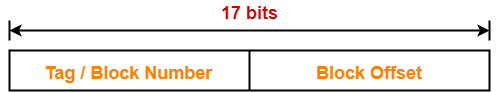### Number of Bits in Block Offset-

We have,

Block size

= 256 bytes

= 28 bytes

Thus, Number of bits in block offset = 8 bits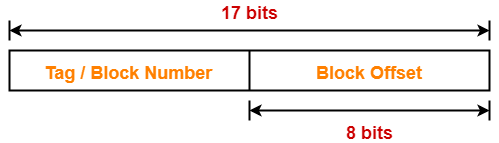### Number of Bits in Tag-

Number of bits in tag

= Number of bits in physical address – Number of bits in block offset

= 17 bits – 8 bits

= 9 bits

Thus, Number of bits in tag = 9 bits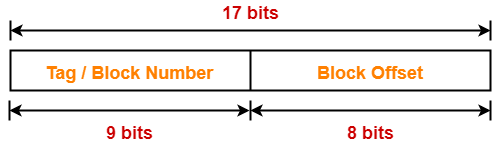### Number of Lines in Cache-

Total number of lines in cache

= Cache size / Line size

= 16 KB / 256 bytes

= 214 bytes / 28 bytes

= 26 lines

### Tag Directory Size-

Tag directory size

= Number of tags x Tag size

= Number of lines in cache x Number of bits in tag

= 26 x 9 bits

= 576 bits

= 72 bytes

Thus, size of tag directory = 72 bytes

## Problem-02:

Consider a fully associative mapped cache of size 512 KB with block size 1 KB. There are 17 bits in the tag. Find-

1. Size of main memory
2. Tag directory size

## Solution-

Given-

• Cache memory size = 512 KB
• Block size = Frame size = Line size = 1 KB
• Number of bits in tag = 17 bits

We consider that the memory is byte addressable.

### Number of Bits in Block Offset-

We have,

Block size

= 1 KB

= 210 bytes

Thus, Number of bits in block offset = 10 bits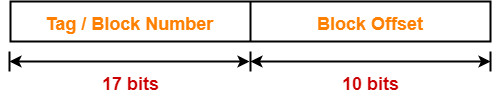### Number of Bits in Physical Address-

Number of bits in physical address

= Number of bits in tag + Number of bits in block offset

= 17 bits + 10 bits

= 27 bits

Thus, Number of bits in physical address = 27 bits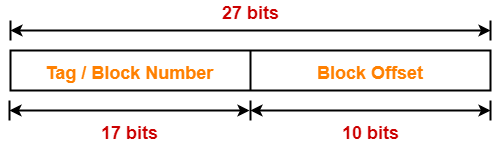### Size of Main Memory-

We have,

Number of bits in physical address = 27 bits

Thus, Size of main memory

= 227 bytes

= 128 MB

### Number of Lines in Cache-

Total number of lines in cache

= Cache size / Line size

= 512 KB / 1 KB

= 512 lines

= 29 lines

### Tag Directory Size-

Tag directory size

= Number of tags x Tag size

= Number of lines in cache x Number of bits in tag

= 29 x 17 bits

= 8704 bits

= 1088 bytes

Thus, size of tag directory = 1088 bytes

## Problem-03:

Consider a fully associative mapped cache with block size 4 KB. The size of main memory is 16 GB. Find the number of bits in tag.

## Solution-

Given-

• Block size = Frame size = Line size = 4 KB
• Size of main memory = 16 GB

We consider that the memory is byte addressable.

### Number of Bits in Physical Address-

We have,

Size of main memory

= 16 GB

= 234 bytes

Thus, Number of bits in physical address = 34 bits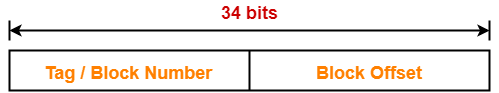### Number of Bits in Block Offset-

We have,

Block size

= 4 KB

= 212 bytes

Thus, Number of bits in block offset = 12 bits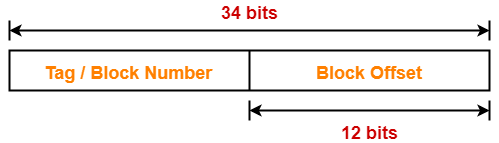### Number of Bits in Tag-

Number of bits in tag

= Number of bits in physical address – Number of bits in block offset

= 34 bits – 12 bits

= 22 bits

Thus, Number of bits in tag = 22 bits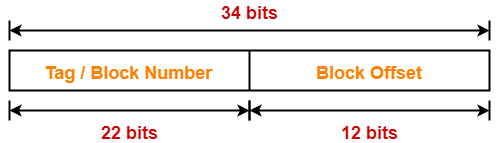To watch video solutions and practice more problems,

Watch this Video Lecture

Next Article- Set Associative Mapping | Implementation & Formulas

Get more notes and other study material of Computer Organization and Architecture.

Watch video lectures by visiting our YouTube channel LearnVidFun.